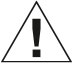# Kids First Math: Base Ten Blocks Math Kit with Activity Cards

\$29.95
SKU: 568005
##### Product Description

Time after time, experience shows that learning by manipulating physical objects with one’s own hands — learning by doing things firsthand, rather than being told about them or reading about them in a book — is extremely effective. Thames & Kosmos has a long history of incorporating this principle into science education. Now, we are bringing this experience to the Kids First Math line, a series of nine hands-on kits with activity cards or lesson guides designed to demonstrate and teach concepts in mathematics, as well as other skills such as logical thinking, problem solving, and creative thinking.

Kids First Math: Base Ten Blocks Math Kit with Activity Cards

+ A set of 121 plastic connecting blocks representing 2,200 units (1 cubic centimeter each)

+ Used to teach the base ten concepts, the decimal system, area, volume, and basic mathematical operations

+ Also develops skills in spatial intelligence and fine motor skills

+ Visual and hands-on math learning

+ Durable plastic for long-term use and easy cleaning

+ Includes 100 1-cm cubes, 10 10x1x1-cm rods, 10 10x10x1-cm squares, 1 10x10x10-cm cube, 5 activity cards, plastic storage boxWARNING: CHOKING HAZARD — Small parts. Not for children under 3 yrs.
##### Product Information

Ages: 4+
Experiments: 10
Piece Count: 126
Manual Pages: 10
Product Dimensions: 11 x 4.1 x 6.7 in.
Product Weight: 2.1 lbs
Manual Dimensions: 5.8 x 4.1 in.
Batteries Required: N/A
Country of Origin: Taiwan
Year Released: 2021

##### CCSS Correlations

K.CC.A.1 Know number names and the count sequence.

K.CC.A.2 Know number names and the count sequence.

K.CC.B.4 Count to Tell the Number of Objects

K.CC.B.5 Count to Tell the Numbers of Objects

K.CC.C.6 Compare Numbers

K.OA.A.1 Understand addition as putting together and adding to, and understand subtraction as taking apart and taking from.

K.OA.A.2 Understand addition as putting together and adding to, and understand subtraction as taking apart and taking from.

K.OA.A.3 Understand addition as putting together and adding to, and understand subtraction as taking apart and taking from.

K.OA.A.4 Understand addition as putting together and adding to, and understand subtraction as taking apart and taking from.

K.OA.A.5 Understand addition as putting together and adding to, and understand subtraction as taking apart and taking from.

K.NBT.A.1 Work with numbers 11-19 to gain foundations for place value.

1.OA.A.1 Represent and solve problems involving addition and subtraction.

1.OA.B.3 Understand and apply properties of operations and the relationship between addition and subtraction.

1.OA.B.4 Understand and apply properties of operations and the relationship between addition and subtraction.

1.OA.C.6 Add and subtract within 20.

1.OA.D.7 Work with addition and subtraction equations.

1.OA.D.8 Work with addition and subtraction equations.

1.NBT.A.1 Extend the counting sequence.

1.NBT.B.2 Understand place value.

1.NBT.B.3 Understand place value.

1.NBT.C.4 Use place value understanding and properties of operations to add and subtract.

1.NBT.C.6 Use place value understanding and properties of operations to add and subtract.

2.OA.C.3 Work with equal groups of objects to gain foundations for multiplication.

2.OA.C.4 Work with equal groups of objects to gain foundations for multiplication.